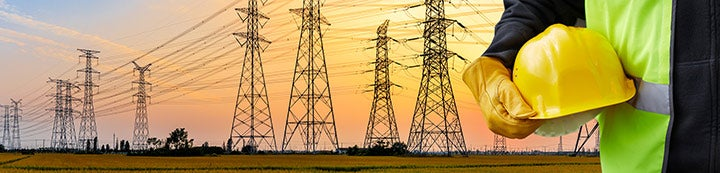1 - 3 of 3 Posts

#### owl

·
##### Registered
Joined
·
364 Posts
Discussion Starter · ·
It says:

A device or utilization equipment wider than a single 50 mm (2 in.) device box as described in Table 314.16(A) shall have double volume allowances provided for each gang required for mounting.

My interpretation:

For big devices that take up more than one gang, count a double volume for each gang the box takes up. For example, here is my box fill calculation for a box containing a receptacle for a 30 A 120/240 electric dryer that takes up two gangs. Nothing else is in the box:

3 #10 conductors @ 2.50 cubic inches each = 7.5
EGC = 2.5
dryer receptacle = one double volume for each of the two gangs = (2.5+2.5) + (2.5+2.5) = 10

7.5 + 2.5 + 10 = 20 cubic inches

I know we just put them in a double gang at work, but I'm trying to understand.

#### HARRY304E

·
##### RIP 1959-2015
Joined
·
39,618 Posts
It says:

A device or utilization equipment wider than a single 50 mm (2 in.) device box as described in Table 314.16(A) shall have double volume allowances provided for each gang required for mounting.

My interpretation:

For big devices that take up more than one gang, count a double volume for each gang the box takes up. For example, here is my box fill calculation for a box containing a receptacle for a 30 A 120/240 electric dryer that takes up two gangs. Nothing else is in the box:

3 #10 conductors @ 2.50 cubic inches each = 7.5
EGC = 2.5
dryer receptacle = one double volume for each of the two gangs = (2.5+2.5) + (2.5+2.5) = 10

7.5 + 2.5 + 10 = 20 cubic inches

I know we just put them in a double gang at work, but I'm trying to understand.
..........

That's what we all do:thumbsup:

These are good if you doing old work..http://t.homedepot.com/p/Carlon-2-G..._pip1_rr-1-_-NA-_-100315472-_-N&showPLP=false

#### Deep Cover

·
##### Registered
Joined
·
2,923 Posts
You are correct, however, a dryer receptacle is a bad example because it can mount to a single gang box or a 2/G box.

•owl
1 - 3 of 3 Posts